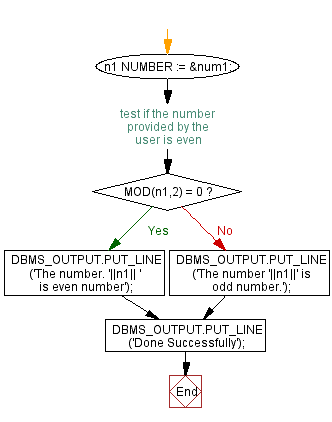﻿ PL/SQL Control Statement: Check whether a number is even or odd. - w3resource# PL/SQL Control Statement Exercises: Check whether a number is even or odd

## PL/SQL Control Statement: Exercise-3 with Solution

Write a PL/SQL program to check whether a number is even or odd.

Sample Solution:

PL/SQL Code:

``````DECLARE
n1 NUMBER := &num1;
BEGIN
-- test if the number provided by the user is even
IF MOD(n1,2) = 0 THEN
DBMS_OUTPUT.PUT_LINE ('The number. '||n1||
' is even number');
ELSE
DBMS_OUTPUT.PUT_LINE ('The number '||n1||' is odd number.');
END IF;
DBMS_OUTPUT.PUT_LINE ('Done Successfully');
END;
/
``````

Sample Output:

```Enter value for num1: 19
old   2: n1 NUMBER := &num1;
new   2: n1 NUMBER := 19;
The number 19 is odd number.
Done Successfully

PL/SQL procedure successfully completed.
```

Flowchart:Improve this sample solution and post your code through Disqus

What is the difficulty level of this exercise?

﻿